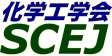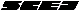## \$B9V1i%W%m%0%i%`!J2q>l!&F|DxJL!K(B

### O\$B2q>l(B \$BBh(B3\$BF|(B

\$B:G=*99?7F|;~!'(B2012-08-20 15:02:17
\$B9V1i(B
\$B;~9o(B
\$B9V1i(B
\$BHV9f(B
\$B9V1iBjL\!?H/I=\$B%-!<%o!<%I(B\$BJ,N`(B
\$BHV9f(B
\$B\$BHV9f(B
\$B%7%s%]%8%&%`(B \$B!c2=3XAuCV\$K\$*\$1\$k:`NA5;=Q!d(B
(9:00\$B!A(B9:40)\$B!!(B(\$B;J2q(B \$B
9:00\$B!A(B 9:40O301[\$B>7BT9V1i(B]\$B%_%/%m\$*\$h\$S%^%/%mM;9gNO3X\$K4p\$E\$/@h?JBQG.:`NA\$N%/%j!<%W
(\$BElKLBg(B) \$B!{2#KY(B \$BTh8w(B
Creep Fracture Life
Creep Damage
Creep Crack
S-41624
(9:40\$B!A(B11:00)\$B!!(B(\$B:BD9(B \$B5WJ]Fb(B \$B>;IR(B)
9:40\$B!A(B 10:00O303\$B?e>x5\$2~
(\$B@iBeED2=9)7z@_(B) \$B!{(B(\$B@5(B)\$B
Creep Damage
Remaining Life Estimation
Heat Resisting Cast Alloy
S-41198
10:00\$B!A(B 10:20O304RBM(\$B%j%9%/%Y!<%9%a%s%F%J%s%9(B)\$BE,MQJ}K!\$N9M\$(J}\$H%=%U%H%&%'%"\$N9=C[(B
(IHI\$B%^%j%s(B) \$B!{BgJ?(B \$B6);K(B \$B!&(B (IHI) \$BIY;N(B \$B>4IW(B \$B!&(B (IHI\$B%^%j%s(B) \$B!5,(B \$B!&(B \$B;VB<(B \$B5A<#(B \$B!&(B (\$B%Y%9%H%^%F%j%"(B) \$BLZ86(B \$B=E8w(B
RBM
Maintenance
Inspection
S-41552
10:20\$B!A(B 10:40O305\$BN3;R>WFM8=>]\$r1~MQ\$7\$?9b294D6-2<\$N%;%i%_%C%/%3!<%F%#%s%0:`NA\$N5!3#E*@-
(\$B9-Bg1!9)(B) \$B!{(B(\$B@5(B)\$Bc&K\(B \$BNIB'(B \$B!&(B (\$B@5(B)\$B8eF#(B \$Bb+Fs(B
ceramic coating materials
mechanical properties
measurements
S-41246
10:40\$B!A(B 11:00O306\$B%"%k%_(B-\$B9]HD\$NBQ?)@-I>2AK!\$N8!F\$(B
(\$B9-Bg1!9)(B) \$B!{(B(\$B@5(B)\$Bc&K\(B \$BNIB'(B \$B!&(B \$B_7ED(B \$BOB1Q(B \$B!&(B \$B:#N\$(B \$B2m;J(B \$B!&(B (\$B%^%D%@(B) \$B@>8}(B \$B>!Li(B
galvanized steel
corrosion
evaluation
S-41215
(11:00\$B!A(B12:00)\$B!!(B(\$B:BD9(B \$Bc&K\(B \$BNIB'(B)
11:00\$B!A(B 11:20O307Corrosion rate of metals in rubber seed oil
(\$B%Z%H%m%J%99)Bg(B) \$B!{(B(\$B@5(B)\$B>eB<(B \$BK';0(B \$B!&(B Basha Hafif \$B!&(B (\$B@5(B)Osman Noridah
corrosion rate
metal
rubber seed oil
S-41262
11:20\$B!A(B 11:40O308\$BN2;@5\$AjIt\$XK=O*\$7\$?%S%K%k%(%9%F%k
(\$BEl9)Bg(B) \$B!{(B(\$B3X(B)\$B9b66(B \$B91G7(B \$B!&(B (\$B@5(B)\$B@DLZ(B \$B:M;R(B \$B!&(B (\$B@5(B)\$B5WJ]Fb(B \$B>;IR(B
gas phase
sulfuric acid
S-41684
11:40\$B!A(B 12:00O309FRP\$B@=CyAe5!4o\$N\$?\$a\$N%"%s%A%(!<%8%s%09=B\$(B
(\$BEl9)Bg(B) \$B!{(B(\$B@5(B)\$B5WJ]Fb(B \$B>;IR(B \$B!&(B \$B;wFb(B \$BM\$Je(B \$B!&(B (\$B@5(B)\$B@DLZ(B \$B:M;R(B \$B!&(B (\$BF|Bg@8;:9)(B) (\$B@5(B)\$B
FRP storage
smart structure
diffusion
S-41648

\$B9V1i%W%m%0%i%`(B
\$B2=3X9)3X2q(B \$BBh(B44\$B2s=)5(Bg2q(B(C) 2012 \$B8x1W
Most recent update: 2012-08-20 15:02:17
For more information contact \$B2=3X9)3X2qElKL;YIt(B \$BBh(B44\$B2s=)5(Bg2q(B \$BLd\$\$9g\$;78(B
E-mail: inquiry-44fwww3.scej.org
This page was generated byeasp 2.28; proghtml 2.28c (C)1999-2011 kawase